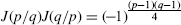## 12.5 WILLIAMS VARIATION OF RSA

e = 2 is not a permissible exponent in RSA, as the mapping Ee is not one-to-one. The ambiguity in decipherment might be resolved by using a standard format for the plaintext x. It is unlikely, but not proved that only one of the (four) square roots would be in the standard format.

Hugh Williams  found a very clever way around this difficulty. He transforms the plaintext x into E1[x] where E1 is an invertible mapping with inverse D1. For primes with appropriate restrictions, there exist transformations E2 and D2 that correspond to encipherment and encipherment such thatThe Jacobi symbol J(p/q) when q is a prime is defined byJ(p/q) can be extended for q not being a prime.

Proposition 12.8: (Properties of the Jacobi Symbol):

12.8a If p1 = p2 (modulo q), then J(p1/q) = J(p2/q);

12.8b J(p1p2/q) = J(p1/q)J(p2/q);

12.8c J(p/q1q2) = J(p/q2)J(p/q2);

12.8dif p, q are odd; and

12.8e If q is a prime, then.

Proposition 12.9:  If p, q are primes such that 3 = p (modulo 4) = q (modulo 4) and J(x/pq) = 1, then

Proof: Using Proposition 12.8c and the hypothesis ...

Get Computer Security and Cryptography now with the O’Reilly learning platform.

O’Reilly members experience live online training, plus books, videos, and digital content from nearly 200 publishers.